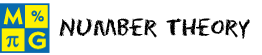# Number TheoryThis page lists the Learning Objectives for all lessons in Unit 3.

#### Factors and Greatest Common Factors

The student will be able to:

• Define factor, whole number, counting number, remainder, quotient and greatest common factor.
• Connect the whole-number dimensions of a rectangular garden to finding factors.
• Identify and list all factors of a given whole number.
• Determine the greatest common factor of two or more whole numbers.
• Describe the procedure for finding the greatest common factor of two or more whole numbers.
• Recognize the difference between a common factor and the greatest common factor of two or more whole numbers.
• Apply factoring concepts and procedures to complete five interactive exercises.

#### Multiples and Least Common Multiples

The student will be able to:

• Define multiple and least common multiple.
• Identify and list the set of multiples for a given whole number.
• Determine the least common multiple of two or more whole numbers.
• Recognize that the set of multiples for a given whole number is infinite.
• Describe the procedure for finding the least common multiple of two or more whole numbers.
• Recognize the difference between a common multiple and the least common multiple of two or more whole numbers.
• Apply multiple concepts and procedures to complete five interactive exercises.

#### Prime and Composite Numbers

The student will be able to:

• Define prime, composite and inclusive.
• Determine if a given whole number is prime or composite by finding all of its factors.
• Describe the procedure for determining if a whole number is prime or composite.
• Identify and list all prime numbers within a given range of whole numbers.
• Recognize that the number one is neither prime nor composite.
• Explain why a given number is prime or composite using full sentences.
• Apply prime and composite concepts and procedures to complete five interactive exercises.

#### Divisibility Tests

The student will be able to:

• Define divisibility test.
• Restate divisibility tests for 2, 3, 4, 5, 6, 8, 9 and 10.
• Perform a single divisibility test on a given whole number.
• Perform several divisibility tests on a given whole number.
• Choose and perform divisibility tests to determine if a number is prime or composite.
• Recognize the need for divisibility tests when working with large, whole numbers.
• Choose and perform divisibility tests to complete five interactive exercises.

#### Exponents

The student will be able to:

• Define base and exponent.
• Discover the need for exponential notation when writing a product of many factors.
• Recite and write numbers in exponential form.
• Indicate if a number is written in exponential form, factor form or standard form.
• Restate the rules for a base with an exponent of zero and one.
• Convert numbers between exponential form, factor form and standard form.
• Apply exponential concepts to complete five interactive exercises.

#### Pattern and Exponents

The student will be able to:

• Define powers.
• Identify the rule for a pattern of numbers by examining a list of powers.
• Predict the next number in the list of powers for a given a number.
• Given a base raised to the nth power, determine the standard form of that base raised to the n+1 power using a single multiplication.
• Evaluate five interactive exercises with word problems.
• Analyze each word problem to identify the given information.
• Formulate a strategy for solving each problem.
• Apply advanced exponential concepts to solve problems.

#### Practice Exercises

The student will be able to:

• Examine ten interactive exercises for all topics in this unit.
• Determine which concepts and procedures are needed to complete each practice exercise.
• Compute answers by applying procedures, and by predicting the next number in a sequence.
• Self-assess knowledge and skills acquired from this unit.

#### Challenge Exercises

The student will be able to:

• Evaluate ten interactive exercises with word problems for all topics in this unit.
• Analyze each word problem to identify the given information.
• Formulate a strategy for solving each problem.
• Apply strategies to solve routine and non-routine problems.
• Synthesize all information presented in this unit.
• Connect number theory to the real world.
• Develop problem-solving skills.

#### Solutions

The student will be able to:

• Examine the solution for each exercise presented in this unit.
• Identify which solutions need to be reviewed.
• Compare solutions to completed exercises.
• Identify and evaluate incorrect answers.
• Amend and label original answers.
• Identify areas of strength and weakness.
• Decide which concepts and procedures need to be reviewed from this unit.

### Order your CD today for Home or SchoolOR